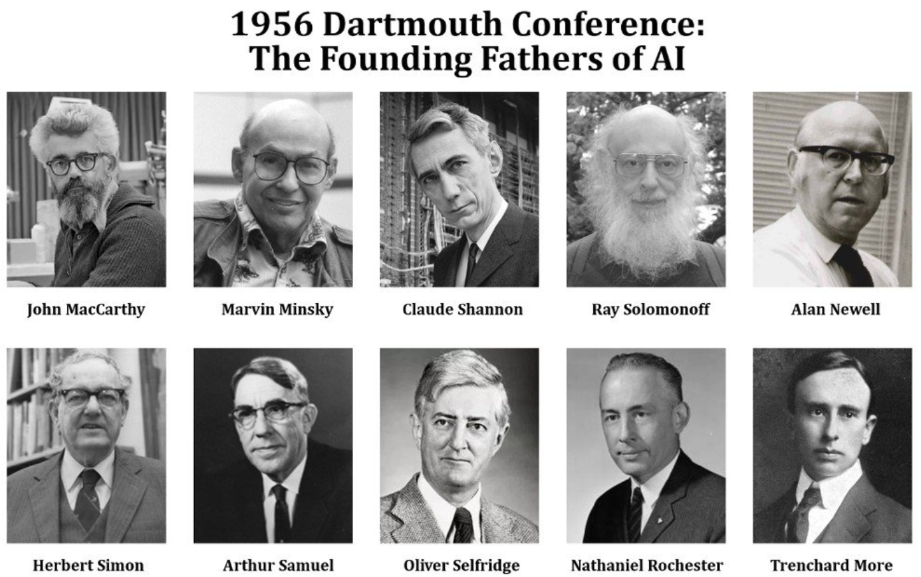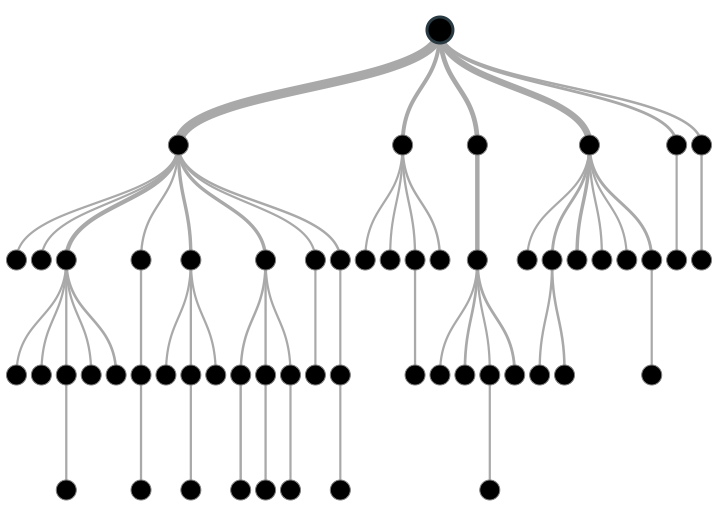# The First Wave of AI: Reasoning as Search

This post is part of a series.

In 1955, scientists Newell, Simon, and Shaw created a computer program to re-prove theorems in the Principia Mathematica, a landmark book on mathematical logic. The program, called the Logic Theorist, succeeded in proving an impressive 38 of the first 52 theorems, and even found some new and more elegant proofs.

Newell and Simon presented their Logic Theorist at the 1956 Dartmouth conference, widely considered the birth of AI as a scientific discipline. It was not long until more AI programs had been developed to accomplish other impressive tasks such as solving high school algebra and geometry problems, including word problems.Many of these early AI programs leveraged the same underlying algorithm, reasoning as search, which likened the act of reasoning to the task of searching through a maze of actions for a sequence that would achieve the desired end goal.For example, to prove the simple statement “if a number ends in 8, then it is even” given a set of valid rules, a reasoning-by-search program would construct a maze by forking a path for each possible conclusion that could be made from the assumption that a number ends in 8. Then, for each of those initial conclusions, another set of paths would be forked, consisting of the next round of conclusions that could be made by applying a rule to the initial conclusion. Somewhere in the maze would reside the conclusion that the number is even, and the computer would search all the possible paths through the maze until it found the shortest path leading to that particular conclusion. The path, comprising a chain of conclusions, would then form a proof: “if a number ends in 8, then is divisible by 2, and thus it is even.”

To solve more complex problems, reasoning as search would sometimes require checking an enormous number of paths, sometimes too many even for a computer to complete in a reasonable amount of time. To decrease the number of paths that needed to be checked, researchers would often employ various heuristics or “rules of thumb” to ignore pathways that were unlikely to lead to a solution.

Excited by the initial accomplishments of early AI, researchers predicted that a general AI would be built in less than 20 years, and government agencies like DARPA jumped to fund further research.

But researchers and funders alike were blind to an issue that would become a major bottleneck in the growth of AI: a lack of computation power. Computers of the 1960s did not have enough memory or processing speed to run AI programs at the scale needed to accomplish anything truly useful, and as a result, researchers were unable to achieve the overhyped predictions that they had made to their funders.

In response, funding was withdrawn and AI research stalled in what would become known as the AI winter of the 1970s.

This post is part of a series.

Tags: